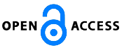Isaac Scientific Publishing### Decompositions of Symmetry Using Odds-Symmetry for Square Contingency Tables

Download PDF (441.4 KB) PP. 23 - 30 Pub. Date: September 1, 2018

### Author(s)

• Shuji Ando*
Department of Information and Computer Technology, Faculty of Engineering, Tokyo University of Science, Katsushika-ku, Tokyo, 125-8585, Japan
• Kyohei Aoba
Department of Information and Computer Technology, Faculty of Engineering, Tokyo University of Science, Katsushika-ku, Tokyo, 125-8585, Japan

### Abstract

For square contingency tables, many decompositions of the symmetry model were given. If the symmetry model does not hold, the decomposition of symmetry is useful to analyze the cause that the symmetry model fits poorly. The present paper shows the decomposition of the symmetry model using odds-symmetry, and the test statistic for the symmetry model is equal to the sum of those for decomposed models. This paper also gives the decomposition of the conditional symmetry model using odds-symmetry. By comparing the existing decompositions of the symmetry model, we show that the proposed decompositions are useful.

### Keywords

Marginal symmetry, ordinal category, partitioning, test statistic.

### References

 Bishop, Y. M. M., Fienberg, S. E., and Holland, P. W. (1975). Discrete Multivariate Analysis: Theory and Practice. Cambridge, Massachusetts: The MIT Press.

 Bowker, A. H. (1948). A test for symmetry in contingency tables. Journal of the American Statistical Association, 43, 572-574.

 Caussinus, H. (1965). Contribution à l’analyse statistique des tableaux de corr′elation. Annales de la Facult′e des Sciences de l’Universit′e de Toulouse, 29, 77-182.

 Darroch, J. N. and Silvey, S. D. (1963). On testing more than one hypothesis. Annals of Mathematical Statistics, 34, 555-567.

 Kateri, M, Gottard, A, and Tarantola, C. (2017). Generalised quasi-symmetry models for ordinal contingency tables, Australian & New Zealand Journal of Statistics, 59, 239-253.

 McCullagh, P. (1978). A class of parametric models for the analysis of square contingency tables with ordered categories. Biometrika, 65, 413-418.

 Read, C. B. (1977). Partitioning chi-square in contingency tables: A teaching approach. Communications in Statistics - Theory and Methods, 6, 553-562.

 Stuart, A. (1955). A test for homogeneity of the marginal distributions in a two-way classification. Biometrika, 42, 412-416.

 Tahata, K. and Tomizawa, S. (2014). Symmetry and asymmetry models and decompositions of models for contingency tables. SUT Journal of Mathematics, 50, 131-165.

 Tominaga, K. (1979). Nippon no Kaisou Kouzou (Japanese Hierarchical Structure). University of Tokyo press, Tokyo (in Japanese).

 Tomizawa, S. (1984). Three kinds of decompositions for the conditional symmetry model in a square contingency table. Journal of the Japan Statistical Society, 14, 35-42.

 Tomizawa, S. (1985). Decompositions for odds-symmetry models in a square contingency table with ordered categories. Journal of the Japan Statistical Society, 15, 151-159.

 Tomizawa, S., and Tahata, K. (2007). The analysis of symmetry and asymmetry: orthogonality of decomposition of symmetry into quasi-symmetry and marginal symmetry for multi-way tables. Journal de la Soci′et′e Fran?aise de Statistique, 148, 3-36.